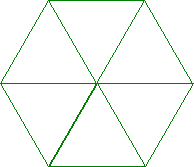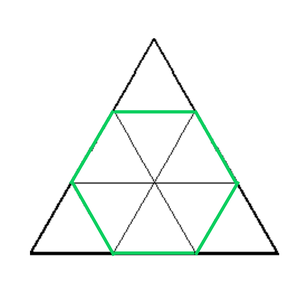Open in App
Not now

# Count of Equilateral Triangles of unit length possible from a given Hexagon

• Difficulty Level : Expert
• Last Updated : 10 May, 2021

Given an array S[] consisting of the lengths of the 6 sides of a Hexagon, the task is to calculate the number of equilateral triangles of unit length that can be made from the given hexagon.

Examples:

Input: S = {1, 1, 1, 1, 1, 1}
Output:
Explanation:Input: S = {2, 2, 1, 3, 1, 2}
Output: 19
Explanation:Approach: The following observations need to be made to solve the given problem:

• Consider an equilateral triangle of ‘X’ side length. It has been divided into smaller triangles of unit length each, by drawing lines parallel to its sides.
• Below are the images of three such equilateral triangles:

• In each of the above three examples, the count of unit length equilateral triangles possible are:

1. X = 2: 4 equilateral triangles of 1 unit length side.
2. X = 3: 9 equilateral triangles of 1 unit length side.
3. X = 5: 25 equilateral triangles of 1 unit length side.

• By observation, it is clear that, for an equilateral triangle of side length X, X2 equilateral triangles of unit length are possible.
• Extending this observation to Hexagons, inscribe Hexagons inside the equilateral triangles, as shown below:A regular Hexagon inscribed from equilateral triangle of side X = 3, has 6 mini triangles inside it.An irregular Hexagon inscribed from the equilateral triangle of side X = 5, has 19 mini triangles inside it.

• It can be observed that by removing a certain number of mini triangles from the bigger triangle, the hexagon with given dimensions can be found.

The formula for counting the number of triangles of unit length can be generalized for a Hexagon having six sides S1 , S2 , S3 , S4 , S5 , S6 as:

Number of triangles that can be formed = ( S1 + S2 + S3 )2 – S12 – S32 – S52

Below is the implementation of the above approach:

## C++

 `// C++ program to implement``// the above approach``#include ``using` `namespace` `std;` `// Function to calculate the``// the number of Triangles possible``int` `calculateTriangles(``int` `sides[])``{``    ``double` `count = ``pow``(sides + sides +``                       ``sides, 2);``    ``count -= ``pow``(sides, 2);``    ``count -= ``pow``(sides, 2);``    ``count -= ``pow``(sides, 2);``    ` `    ``return` `(``int``)(count);``}` `// Driver Code``int` `main()``{``    ` `    ``// Regular Hexagon``    ``int` `sides[] = { 1, 1, 1, 1, 1, 1 };``    ``cout << (calculateTriangles(sides)) << endl;` `    ``// Irregular Hexagon``    ``int` `sides1[] = { 2, 2, 1, 3, 1, 2 };``    ``cout << (calculateTriangles(sides1)) << endl;``    ` `    ``return` `0;``}` `// This code is contributed by 29AjayKumar`

## Java

 `// Java program to implement``// the above approach``import` `java.util.*;` `class` `GFG{` `// Function to calculate the``// the number of Triangles possible``static` `int` `calculateTriangles(``int` `sides[])``{``    ``double` `count = Math.pow(sides[``0``] + sides[``1``] +``                            ``sides[``2``], ``2``);``    ``count -= Math.pow(sides[``0``], ``2``);``    ``count -= Math.pow(sides[``2``], ``2``);``    ``count -= Math.pow(sides[``4``], ``2``);``    ` `    ``return` `(``int``)(count);``}` `// Driver Code``public` `static` `void` `main(String[] args)``{``    ` `    ``// Regular Hexagon``    ``int` `sides[] = { ``1``, ``1``, ``1``, ``1``, ``1``, ``1` `};``    ``System.out.print((calculateTriangles(sides)) + ``"\n"``);` `    ``// Irregular Hexagon``    ``int` `sides1[] = { ``2``, ``2``, ``1``, ``3``, ``1``, ``2` `};``    ``System.out.print((calculateTriangles(sides1)) + ``"\n"``);``}``}` `// This code is contributed by amal kumar choubey`

## Python3

 `# Python3 Program to implement``# the above approach` `# Function to calculate the``# the number of Triangles possible``def` `calculateTriangles(sides):``    ``count ``=` `pow``( sides[``0``] ``+` `sides[``1``] ``+` `sides[``2``], ``2``)``    ``count ``-``=` `pow``( sides[``0``], ``2``)``    ``count ``-``=` `pow``( sides[``2``], ``2``)``    ``count ``-``=` `pow``( sides[``4``], ``2``)``    ` `    ``return` `int``(count)` `# Driver Code` `# Regular Hexagon``sides ``=` `[``1``, ``1``, ``1``, ``1``, ``1``, ``1``]``print``(calculateTriangles(sides))` `# Irregular Hexagon``sides ``=` `[``2``, ``2``, ``1``, ``3``, ``1``, ``2``]``print``(calculateTriangles(sides))`

## C#

 `// C# program to implement``// the above approach``using` `System;` `class` `GFG{` `// Function to calculate the``// the number of Triangles possible``static` `int` `calculateTriangles(``int` `[]sides)``{``    ``double` `count = Math.Pow(sides + sides +``                            ``sides, 2);``    ``count -= Math.Pow(sides, 2);``    ``count -= Math.Pow(sides, 2);``    ``count -= Math.Pow(sides, 2);``    ` `    ``return` `(``int``)(count);``}` `// Driver Code``public` `static` `void` `Main(String[] args)``{``    ` `    ``// Regular Hexagon``    ``int` `[]sides = { 1, 1, 1, 1, 1, 1 };``    ``Console.Write((calculateTriangles(sides)) + ``"\n"``);` `    ``// Irregular Hexagon``    ``int` `[]sides1 = { 2, 2, 1, 3, 1, 2 };``    ``Console.Write((calculateTriangles(sides1)) + ``"\n"``);``}``}` `// This code is contributed by amal kumar choubey`

## Javascript

 ``

Output:

```6
19```

Time Complexity: O(1)
Auxiliary Space: O(1)

My Personal Notes arrow_drop_up